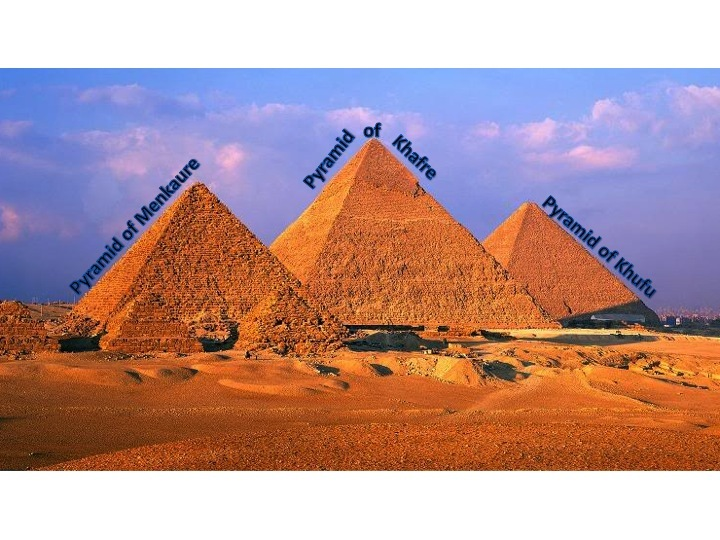# The Great Egyptian Pyramids

Alignments to Content Standards: G-GMD.A.3

Three of the great Egyptian pyramids are pictured below. Each is a square pyramid.1. Calculate the missing information for each the 3 individual pyramids based on the given measurements:

1.  The great Pyramid of Menkaure has a height of about 215 feet and a base side length of about 339 feet. What is its volume?

2.  The great Pyramid of Khafre has a volume of about 74,400,000 cubic feet and a base side length of 706 feet. What is its height?

3.  The great Pyramid of Khufu has a volume of about 86,700,000 cubic feet and a height of 455 feet. What is the length of its base?

2. The Great Pyramid of Khufu once stood 26 feet taller than it is today. Calculate the original volume of the Great Pyramid. Similarly, the Pyramid of Khafre has eroded over time and lost some of its height. If the original volume of the pyramid was approximately 78,300,000 cubic feet, what was its original height?

## IM Commentary

The Great Pyramid of Giza is the oldest monument on the list of the Seven Wonders of the Ancient World. The Great Pyramid of Giza along with the Pyramid of Menkaure and the Pyramid of Khafre were built almost 4600 years ago to protect the bodies and possessions of the pharaohs Khufu, Menkaure, and Khafre. It took 20-25 years to build each pyramid, each with about 2 million blocks of limestone and/or granite.

As one might expect, the Pyramids of Giza do not have integer (linear) measurements; thus, the linear measurements were rounded to the nearest whole number for simplicity and for the volume only three significant digits are used. If the teacher wishes to use the exact measurements to challenge the students, the information may be found through http://www.guardians.net/egypt/pyramids.htm.

The teacher may decide to give the equation for the volume of a pyramid to the students or not. If the equation is given, this task is very easy and could be a quick in-class exercise, or this task could be given with other homework. If the equation is not given, students will most certainly need guidance from the teacher.

The task is aligned to G-GMD.3 at each part involves either finding the volume of a pyramid or using the volume to find the base or height. The standard A-CED.4 is also relevant since this task requires students to rearrange the volume formula for a square pyramid to highlight the relevant quantity (volume, base and height).

## Solution

Since all of the measurements are given to three significant digits, we maintain that level of accuracy throughout the solutions.

1. The volume equation for a square pyramid is $V=\frac{1}{3}bh$, where $b$ is the area of the base and $h$ is the height.

1. For the Pyramid of Menkaure, we are given the height, and can find the area of the base by squaring the side length: $$V = \frac{1}{3}\times 339^2\times 215 \approx 8,240,000 \text{ feet}^3.$$
2. For the Pyramid of Khafre, we are given the volume and the base area (again by squaring the given side length), and are asked to find the height. We can begin by rearranging our volume formula so that $h$ is by itself: Mulitplying both sides by 3 then dividing both sides by $b$ gives us $$h = 3 \times \frac{V}{b}.$$ Substituting the given values for volume and the area of the base we find that the height of Khafre was about 448 feet.

3. For the Great Pyramid of Khufu, we are given the volume and the height, so we need to find the side length of the base. We can once more rearrange our volume, this time highlighting $b$:  Multiplying  both sides by 3 and dividing both sides both sides by $h$ gives $$b = 3 \times \frac{V}{h}.$$ Since $b$ is the area of the square base, we can find the side length of the base by taking the square root: $$\sqrt{b} = \sqrt{\frac{3V}{h}}.$$ Substituting in the given values for $V$ and $h$ we find that $b$ is about 756 feet.

2. For the original Pyramid of Khufu, we need to add 26 feet to 455 feet, the height given in question 1. We get 26 + 455 = 481 feet, the ancient height. Now we just need to calculate the ancient volume using the formula $V = \frac{1}{3}bh$. Substituting in the given value for $b$ and the new value of $h$ we find that the volume of the original Pyramid of Khufu was about 91,600,000 cubic feet.  For the Pyramid of Khafre, we need only evaluate $h=3V/b$ when $V=78,300,000$ cubic feet and the length of the base is $706$ feet, which gives an original height of about 471 feet.